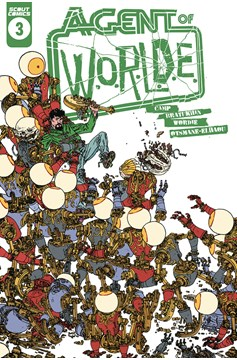•# Agent of W.O.R.L.D.E. #3 (Of 4)

EUR € 5.99

From my secret family to yours: Ex-spies with metal eyes! Happy beginnings and sad endings! Do not observe the cat! Things go from worse to worse! All of that, except in reverse!

## Agent of W.O.R.L.D.E. #3 (Of 4)

Out Of Stock

How much does "Agent of W.O.R.L.D.E. #3 (Of 4)" cost at ?

The price of "Agent of W.O.R.L.D.E. #3 (Of 4)" at is EUR €5.99.

Who is the publisher of "Agent of W.O.R.L.D.E. #3 (Of 4)"?

The publisher of "Agent of W.O.R.L.D.E. #3 (Of 4)" is Scout Comics.

What is the genre of "Agent of W.O.R.L.D.E. #3 (Of 4)"?

The genre of "Agent of W.O.R.L.D.E. #3 (Of 4)" is Science Fiction.

What category does "Agent of W.O.R.L.D.E. #3 (Of 4)" fall under?

"Agent of W.O.R.L.D.E. #3 (Of 4)" falls under the category of Comics.

Who is the artist and cover artist of "Agent of W.O.R.L.D.E. #3 (Of 4)"?

The artist of "Agent of W.O.R.L.D.E. #3 (Of 4)" is Filya Bratukhin. The cover artist is Filya Bratukhin.</span>

Who is the writer of "Agent of W.O.R.L.D.E. #3 (Of 4)"?

The writer of "Agent of W.O.R.L.D.E. #3 (Of 4)" is Deniz Camp.